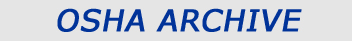## Occupational Safety and Health Administration OSHANOTICE: This is an OSHA Archive Document, and no longer represents OSHA Policy. It is presented here as historical content, for research and review purposes only.

 SilicaOSHA regulates silica exposure using the permissible exposure limit (PEL), which is the maximum amount of airborne dust an employee may be exposed to during a full work shift.

The PEL is dependent on the amount of crystalline silica that is present in the dust. The equation for this calculation is given below. For the most part, OSHA is more concerned with the respirable fraction of the sample because it is more hazardous; however, both respirable and total dust equations are shown. The following parameters are necessary to calculate the PEL and the exposure level.
• % crystalline silica in the air samples (if the laboratory reports the silica concentrations in units of mass convert the mass to percent - e.g., [sample weight (mg) ÷ dust weight (mg) ] × 100% ):
• % quartz
• % cristobalite
• % tridymite
• Total weight of the dust collected in the air samples in milligrams
• Total volume of air sampled for each sample in cubic meters (1000 liters = 1 cubic meter)
• Total sampling time for each air sample in minutes
PEL Equations:
PEL (respirable fraction) = 10 ÷ [% quartz + (% cristobalite × 2) + (% tridymite × 2) + 2]

PEL (total dust) = 30 ÷ [% quartz + (% cristobalite × 2) + (% tridymite × 2) + 2]

The total dust sample is normally taken using the same sampling technique described previously, but without a cyclone.
Exposure Equation:
Exposure = [(mg/m3(1) × time(1)) + (mg/m3(2) × time(2)) + ... + (mg/m3(n) × time(n))] ÷ 480 minutes
Example:
The following example is based on collecting a respirable sample using a cyclone. If a total dust sample were collected (i.e., no cyclone used) the example would only differ in the value used to calculate the PEL.

Two consecutive samples (respirable fraction) from the same employee taken for a combined exposure to silica dusts have the following results:
 Sample Sampling Period (minutes) Total Volume (m3) Weight of Dust (mg) Concentration (mg/m3) Laboratory Results (%) A 238 0.405 0.855 2.1 5.2 quartz 2.3 cristobalite ND tridymite B 192 0.326 0.619 1.9 4.8 quartz 1.7 cristobalite ND tridymite Total 430 0.731 1.474 ND = not detectable

Step 1. Calculate the percentage of quartz, cristobalite, and tridymite in the particulate collected.
Quartz: [5.2% × (0.855/1.474)] + [4.8% × (0.619/1.474)] = 3.0 + 2.0 = 5.0%
Cristobalite: [2.3% × (0.855/1.474)] + [1.7% × (0.619/1.474)] = 1.3 + 0.7 = 2.0%
Tridymite: none detected = 0%
Step 2. Calculate the PEL for the mixture.
PEL(mixture) = 10 mg/m3 ÷ [% quartz + (% cristobalite × 2) + (% tridymite × 2) + 2]
= 10 ÷ [5 + (2.0 × 2) + ( 0 × 2) + 2] = 10 ÷ 11.0 = 0.91 mg/m3
Step 3. Calculate the employee's exposure (8 hour TWA).
Exposure = [(mg/m3(1) × time(1)) + (mg/m3(2) × time(2)) + ... + (mg/m3(n) × time(n))] ÷ 480 minutes
= [(2.1 × 238) + (1.9 × 192)] ÷ 480 = 1.8 mg/m3
Step 4. Calculate the severity of the exposure.
Severity = 1.8 mg/m3 ÷ 0.91 mg/m3 = 2.0
 Silica

### Thank You for Visiting Our Website

#### You are exiting the Department of Labor's Web server.

The Department of Labor does not endorse, takes no responsibility for, and exercises no control over the linked organization or its views, or contents, nor does it vouch for the accuracy or accessibility of the information contained on the destination server. The Department of Labor also cannot authorize the use of copyrighted materials contained in linked Web sites. Users must request such authorization from the sponsor of the linked Web site. Thank you for visiting our site. Please click the button below to continue.

Close